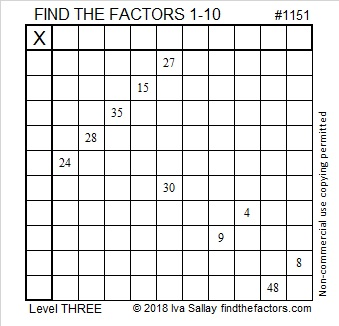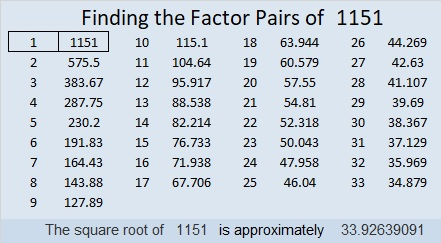# 1151 and Level 3

If you know the common prime factor for 27 and 30, then you can at least start this puzzle. If you work down the first column cell by cell using logic, you should be able to solve the puzzle, too. Good luck!Print the puzzles or type the solution in this excel file: 10-factors-1148-1160

Here are some facts about the number 1151:

• 1151 is a prime number.
• Prime factorization: 1151 is prime.
• The exponent of prime number 1151 is 1. Adding 1 to that exponent we get (1 + 1) = 2. Therefore 1151 has exactly 2 factors.
• Factors of 1151: 1, 1151
• Factor pairs: 1151 = 1 × 1151
• 1151 has no square factors that allow its square root to be simplified. √1151 ≈ 33.92639

How do we know that 1151 is a prime number? If 1151 were not a prime number, then it would be divisible by at least one prime number less than or equal to √1151 ≈ 33.9. Since 1151 cannot be divided evenly by 2, 3, 5, 7, 11, 13, 17, 19, 23, 29 or 31, we know that 1151 is a prime number.1151 is the sum of consecutive primes three different ways:
It is the sum of the twenty-three prime numbers from 7 to 101.
223 + 227 + 229 + 233 + 239 = 1151 and
379 + 383 + 389 = 1151

OEIS.org states that 1151 is the smallest number that is the sum of consecutive prime numbers four different ways, I think they must be considering 1151 = 1151 to be one of those ways.

1151 is palindrome 1L1 in BASE 25 (L is 21 base 10)
because 25² + 21(25) + 1 = 1151

This site uses Akismet to reduce spam. Learn how your comment data is processed.